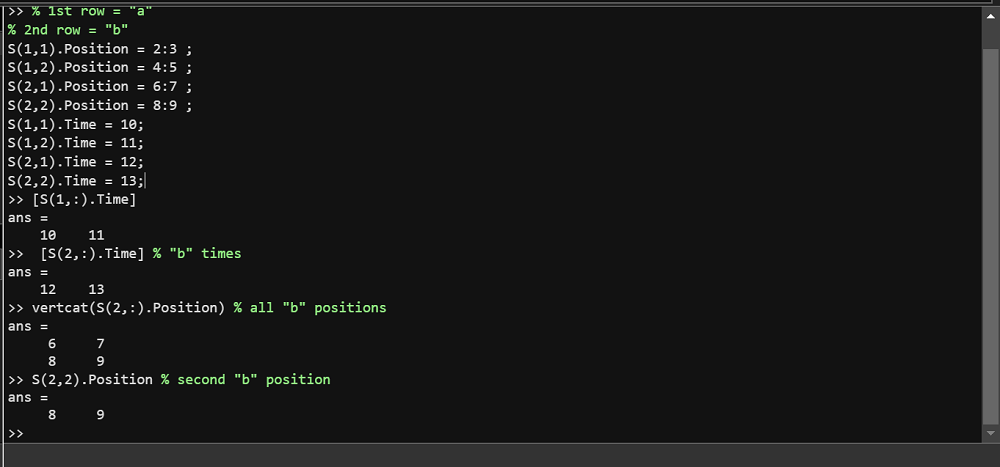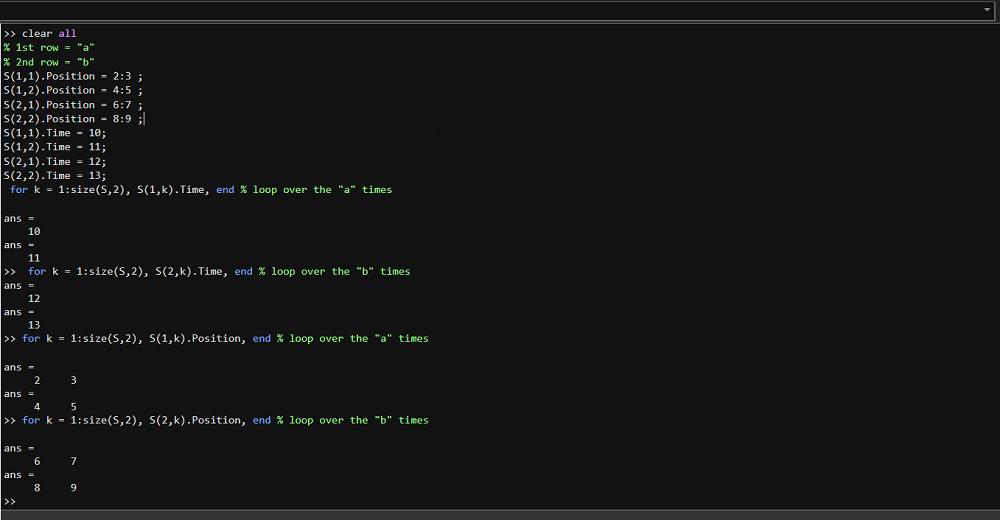Open in App
Not now

# How to Iterate Through Multiple Structures in MATLAB?

• Last Updated : 12 Dec, 2022

In this, we will iterate multiple structures in Matlab. So, let if you want to iterate the multiple structures so simply create one non-scalar structure and iterate with that. Below is the syntax to iterate with the for loop.

#### Syntax:

for i = 1:5

mystruct(i).test = …

mystruct(i).data = …

end

The above method is much faster than accessing separate variables. There are some other methods also and you can use any. Below is an example to iterate multiple structures:-

Example 1:

## Matlab

 `%% clear all``%% 1st row = "a"``%% 2nd row = "b"``S(1,1).Position = 2:3 ;``S(1,2).Position = 4:5 ;``S(2,1).Position = 6:7 ;``S(2,2).Position = 8:9 ;``S(1,1).Time = 10;``S(1,2).Time = 11;``S(2,1).Time = 12;``S(2,2).Time = 13;`

Output:### Explanation:

Above we created structures and in the below, we are taking all:a” row and “b” row positions and you can see the answer in the above output image.

### By Loop:

You can see indexing can be used to access any element of the structure. You can iterate over the structure in any way you want, you can use for loop also to iterate time and position. Let’s see the above example once again:-

#### Syntax:

for k = 1:size(S,2), S(1,k).Time, end % loop over the “a” times

Example 2:

## Matlab

 `% clear all``% 1st row = "a"``% 2nd row = "b"``S(1,1).Position = 2:3 ;``S(1,2).Position = 4:5 ;``S(2,1).Position = 6:7 ;``S(2,2).Position = 8:9 ;``S(1,1).Time = 10;``S(1,2).Time = 11;``S(2,1).Time = 12;``S(2,2).Time = 13;`` ` `% loop over the "a" times``for` `k = 1:size(S,2), S(1,k).Time, ``end` ` ` `% loop over the "b" times``for` `k = 1:size(S,2), S(2,k).Time, ``end` ` ` `% loop over the "a" times``for` `k = 1:size(S,2), S(1,k).Position, ``end` ` ` `% loop over the "b" times``for` `k = 1:size(S,2), S(2,k).Position, ``end`

Output:#### Explanation:

” for k= 1:size(S,2), S(1,k).Time; end ” this for loop is used to iterate Time and similarly, you can use this also for Position. In the above for loop iterate, over “a” and “b” time and you can also iterate in the position of all values of “a” and “b”.

My Personal Notes arrow_drop_up